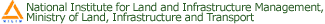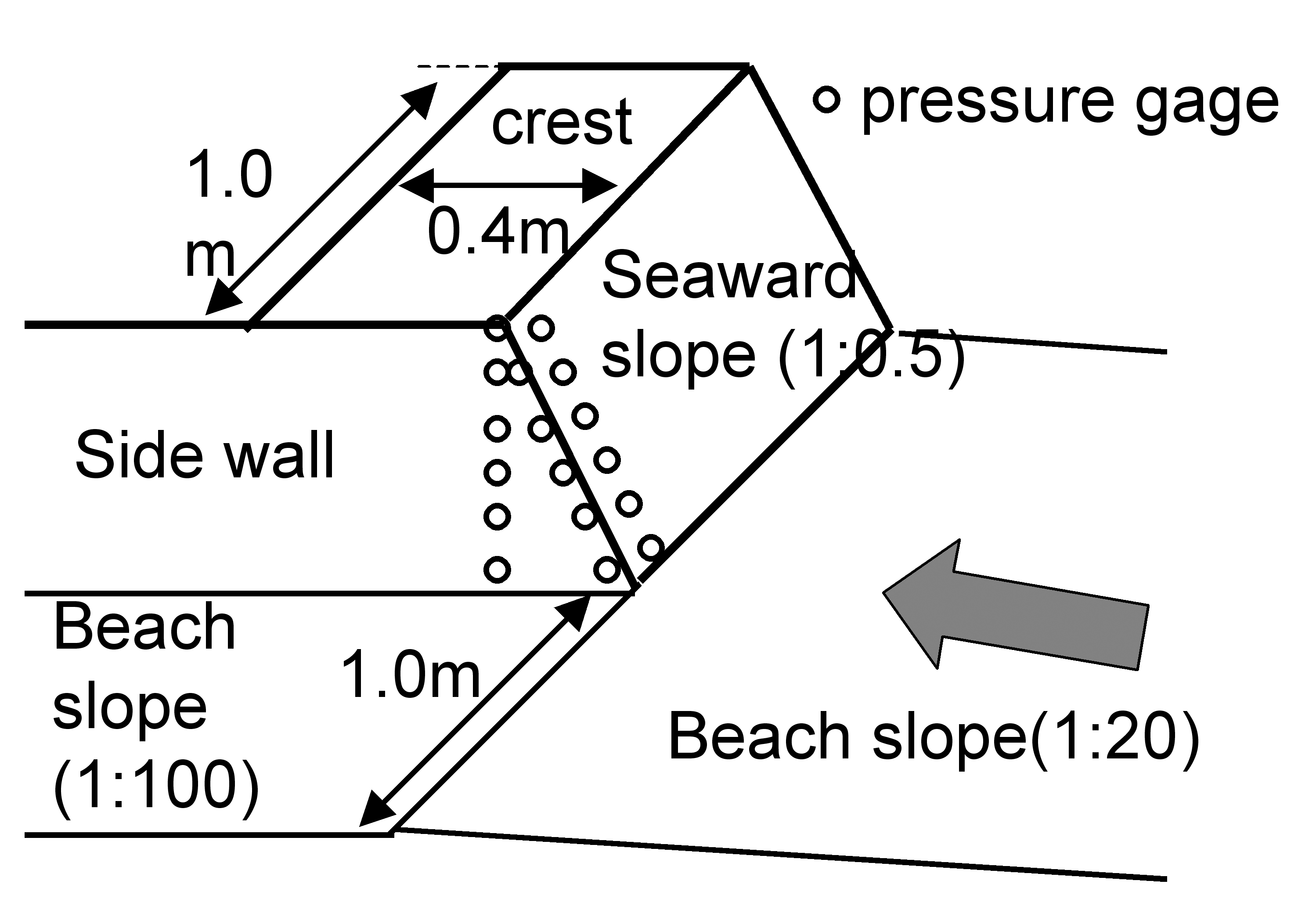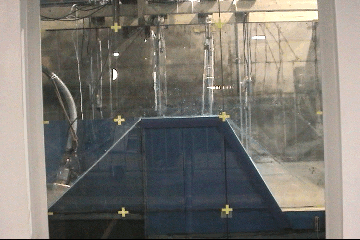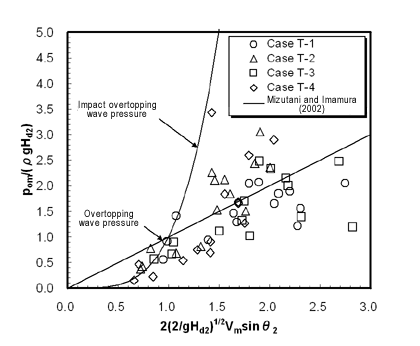Japanese Page# Research themes of the Coast Division  Tsunami/storm surge

## 4. Wave force of tsunami acting on coastal levees

To minimize damage due to tsunami in the inner areas protected by coastal levees, the coastal levees must be stable against the tsunami run-up. The wave force of tsunami is one of the causes of damage to coastal levees, and so a method of investigating it needs to be established. The conventional research is mostly based on model experiments by setting the ocean side of structures to be a horizontal floor. Nevertheless, as coastal levees are generally installed behind a sand beach which is usually inclined, wave force needs to be assessed in consideration of the deformation of run-up waves on such slopes. Thus, large-scale model experiments were conducted in a water channel owned by NILIM to assess the wave force of tsunami acting on levees.

As shown in Figure 1, a slope (sea area: 1/20 gradient, land area: 1/100 gradient) with fixed bed was created in the water channel of 140 m in length, 2 m in width, and 5 m in width, on which a levee model on a 1/10 scale (crest height from the levee foot of 0.5 m, crest width of 0.4 m) was installed. As shown in Table 1, Types 1 to 4, in which the water channel was completely closed, and Type 5, in which half the channel was closed assuming a river mouth, were established in the model.Figure 1 Cross section of the levee model

Table 1 Model dimensionsIn the case of Types 1 to 4, 23 pressure gages were installed at about 0.1-m intervals on the front slope, crest, and back slope of the levee, as well as on the slope behind the levee, as shown in Figure 2. In the case of Type 5, pressure gages were concentrated on the crest and lateral surface of the levee as shown in Figure 3. The sampling frequency of wave pressure was set to be 0.002 s to enable impact wave pressures to be measured, following Mizutani and Imamura (2000). In addition to the pressure gages, capacitance wave gages and current meters were installed at the peripheral corners of the levee, and the overtopping wave discharge was estimated based on the water level measured by the wave gages installed on the landside of the levee.Figure 2 Arrangement of the measurement instruments (Types 1 to 4)Figure 3 Arrangement of the pressure gages (Types 5)

As shown in Table 2, five conditions were established for the tide level in consideration of the existence of the beach in front of the levee. When the tide level was set to be 3.8 m, the shoreline was located at the levee foot. As for Types 1 to 4, the tide level with the five conditions was combined with the solitary wave with three conditions, and 15 different cases of experiments were conducted for these respective models. As for Type 5, on the other hand, three cases of model experiments were conducted as shown in Table 2.

Table 2 Tide level and wave conditionsThe figure below is a video clip for the case when applying a 3.8 m tide level and 0.4 m incident wave height to the Type 3 model. The water level (H4: levee foot, H5: crest top of slope), the current velocity (V5: crest top of slope), and the wave pressure of the front slope (P1: levee foot, P3: 0.2 m upward from the levee foot, P5: 0.4 m upward from the levee foot) of this case are shown in time series in Figure 4. Due to the wave deformation on the slope surface, the wave height reached 1.1 m at H4, and 0.6 m at H5. In addition, the current velocity reached the peak earlier than the water level, and the maximum value exceeded 2 m/s. The maximum value of wave pressure at P1 and P5 was around 100 gf/cm2 (9.8 kPa), but at P3 it exceeded 500 gf/cm2 (49.0 kPa) during 0.004 seconds immediately after the arrival of the run-up wave. Such impact wave pressure was measured in several cases of the model experiments.Tsunami experimentü@Figure 4 Water level, current velocity, and wave pressure on the front slope (Type 3)

1) Wave pressure on the front slope

Figures 5 and 6 show the vertical profile of wave pressure on the front slope. The horizontal axis shows the maximum wave pressure, p, which was nondimensionalized using wave height, H, and other factors at the levee foot, whereas the vertical axis is the ordinate which was nondimensionalized by wave height, H, at the levee foot. The vertical profiles of wave pressures obtained by the calculation formula of Tanimoto et al. (1984) based on a model experiment in which the sinusoidal waves of the initial motion of the uprush of waves crashed against the vertical breakwater, that of Ikeno et al. (2001) based on a model experiment in which a bore due to quick opening of a gate crashed against the vertical wall in the sea area, and that of Ikeno et al. (2003) based on a model experiment in which a bore due to quick opening of a gate crashed against the vertical wall on the land, are also shown in these figures. In the case of 1:1 gradient on the front slope, the wave pressures were smaller than those obtained according to the formula of Tanimoto et al. (1984) in many cases, and the wave pressures in almost all the cases are not underestimated if the formula of Ikeno et al. (2003) is used. In the case of 1:0.5 gradient on the front slope, on the other hand, some of the wave pressures slightly above the still water level are underestimated even using the formula of Ikeno et al. (2003), mainly when the levee foot is superimposed over the shoreline.Figure 5 Vertical profile of wave pressure (1:1 front slope gradient)Figure 6 Vertical profile of wave pressure (1:0.5 front slope gradient)

2) Wave pressure on the back slope and behind the levee

Figure 7 shows the maximum wave pressure, pom, near the toe of the back slope together with a line obtained by the formula of Mizutani and Imamura (2002). The axis of the figure was nondimensionalized by the gravitational acceleration, g, crest height, Hd2, maximum current velocity on the crest, Vm, and gradient of the back slope, āŲ2. Mizutani and Imamura (2002) conducted a model experiment in which a bore flowed over a levee model installed on a horizontal floor, and proposed a formula for calculating the maximum wave pressure behind the levee due to the overflow. As the data obtained by our experiments do not surpass the values obtained by this formula, it is considered that this formula is applicable even in the case of solitary waves.Figure 7 Maximum overtopping wave pressure

References:

Ikeno, M., N. Mori, and H. Tanaka (2001), Experimental Research on the Response and Impact Force of the Wave Force and Drifting Objects due to Breaking Waves, Bores, and Tsunami, Coastal Engineering, JSCE, Vol. 48, pp. 846-850.

Ikeno, M. and H. Tanaka (2003), Experimental Research on Tsunami Running Up on the Land and the Impact Force of Drifting Objects, Coastal Engineering, JSCE, Vol. 50, pp. 721-725.

Tanimoto, K., H. Tsuruya, and S. Nakano (1984), Review of the Tsunami Force and the Cause of Damage to the Bulkhead due to the 1983 Nihonkai-Chubu Earthquake Tsunami, Proc. 31st Japanese Conference on Coastal Engineering, JSCE, pp. 257-261.

Mizutani, S. and F. Imamura (2000), Experiment on the Bore Wave Force Acting on Structures, Coastal Engineering, JSCE, Vol. 47, pp. 946-950.

Mizutani, S. and F. Imamura (2002), Proposal of a Design External Force Calculation Flow in Consideration of the Impact and Overflow of Tsunami Bores, Coastal Engineering, JSCE, Vol. 49, pp. 731-735.

 For inquiries, please contact the Coast Division, River Department, National Institute for Land and Infrastructure Management, Ministry of Land, Infrastructure and TransportMailing address: Asahi Office, 1 Asahi, Tsukuba City, Ibaraki Prefecture, 305-0804 Japan